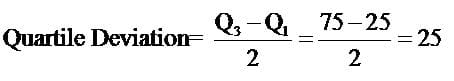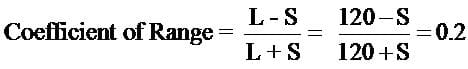Test: Measures Of Dispersion

# Test: Measures Of Dispersion

Test Description

## 10 Questions MCQ Test Economics Class 11 | Test: Measures Of Dispersion

Test: Measures Of Dispersion for Commerce 2022 is part of Economics Class 11 preparation. The Test: Measures Of Dispersion questions and answers have been prepared according to the Commerce exam syllabus.The Test: Measures Of Dispersion MCQs are made for Commerce 2022 Exam. Find important definitions, questions, notes, meanings, examples, exercises, MCQs and online tests for Test: Measures Of Dispersion below.
Solutions of Test: Measures Of Dispersion questions in English are available as part of our Economics Class 11 for Commerce & Test: Measures Of Dispersion solutions in Hindi for Economics Class 11 course. Download more important topics, notes, lectures and mock test series for Commerce Exam by signing up for free. Attempt Test: Measures Of Dispersion | 10 questions in 10 minutes | Mock test for Commerce preparation | Free important questions MCQ to study Economics Class 11 for Commerce Exam | Download free PDF with solutions
 1 Crore+ students have signed up on EduRev. Have you?
Test: Measures Of Dispersion - Question 1

### Quartile deviation with Q1 = 25 and Q3 = 75 is equal to

Detailed Solution for Test: Measures Of Dispersion - Question 1Test: Measures Of Dispersion - Question 2

### Measure of dispersion considering only extreme values is called

Detailed Solution for Test: Measures Of Dispersion - Question 2

Range is the difference between the largest value and the smallest value of a series.
Range = L- S
Here,  L= Largest value, S= Smallest value.
No other values of the series are taken into consideration in this case.

Test: Measures Of Dispersion - Question 3

### The following is a graphic method of measuring dispersion

Detailed Solution for Test: Measures Of Dispersion - Question 3

The departure of the Lorenz curve from the line of equal distribution would indicate the extent of dispersion.

Test: Measures Of Dispersion - Question 4

Lower value of quartile deviation implies

Detailed Solution for Test: Measures Of Dispersion - Question 4

Half of the Inter-Quartile Range is called Quartile Deviation. Lower the value of the quartile deviation, lower the dispersion.

Test: Measures Of Dispersion - Question 5

To measure income inequality we can use

Detailed Solution for Test: Measures Of Dispersion - Question 5

A measure of dispersion can tell us about income inequalities.

Test: Measures Of Dispersion - Question 6

Coefficient of Quartile deviation, with Q1 at 25 and Q3 at 125, equals

Detailed Solution for Test: Measures Of Dispersion - Question 6

The coefficient of quartile deviation (sometimes called the quartile coefficient of dispersion) allows you to compare dispersion for two or more sets of data. The formula is:
Q3-Q1
Q3+Q1
100/150= 0.66

Test: Measures Of Dispersion - Question 7

The relative measures of dispersion are expressed in

Detailed Solution for Test: Measures Of Dispersion - Question 7

The relative measures of dispersion are expressed in percentage. These are used to compare two or more series which are expressed in different units.

Test: Measures Of Dispersion - Question 8

The smallest value of a series, with largest value at 120 and coefficient of range at 0.2, equals

Detailed Solution for Test: Measures Of Dispersion - Question 8S = 80

Test: Measures Of Dispersion - Question 9

The graphical method of studying dispersion is known as

Detailed Solution for Test: Measures Of Dispersion - Question 9

The graphical method of studying dispersion is known as Lorenz curve method. It is named after Dr. Max. O. Lorenz who used it for the first time to measure the distribution of income and wealth.

Test: Measures Of Dispersion - Question 10

The best measure of dispersion is

Detailed Solution for Test: Measures Of Dispersion - Question 10

Standard deviation is the best and widely used measure of dispersion. The properties of standard deviation are:
(i) Standard deviation is rigidly defined.
(ii) It requires harder calculations.
(iii) It depends on all the values of the variable.
(iv) It is based on deviations from the arithmetic mean.
(v) It is capable of further statistical treatment.

## Economics Class 11

200 videos|195 docs|64 tests
 Use Code STAYHOME200 and get INR 200 additional OFF Use Coupon Code
Information about Test: Measures Of Dispersion Page
In this test you can find the Exam questions for Test: Measures Of Dispersion solved & explained in the simplest way possible. Besides giving Questions and answers for Test: Measures Of Dispersion, EduRev gives you an ample number of Online tests for practice

## Economics Class 11

200 videos|195 docs|64 tests